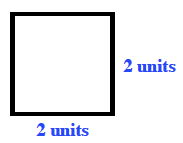### Home > ACC6 > Chapter 7 Unit 7 > Lesson CC1: 7.2.1 > Problem7-46

7-46.

On graph paper, draw your own small design. Use only horizontal and vertical line segments for the sides that lie on the paper’s grid lines. Then draw an enlargement and reduction as described in parts (a) and (b) below.

1. Enlarge your design so that the ratio of lengths of sides is $\frac { 3 } { 1 }$.

Using this ratio, each side should become three times as long.

If you were to enlarge the above square so that each side is three times as long, the labeled sides would each be six units. Try drawing this enlarged square, if you are having trouble.2. Reduce your design so that the ratio of the lengths of sides is $1:2$.

Make each side half the length of the original shape.